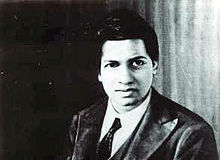Ramanujan can do this instantlySunday, December 22 will be Srinivasa Ramanujan's $126$th birthday. Ramanujan was an amazing mathematician, but one of the things he was most famous for had to do with the number $1729$. When Ramanujan was in the hospital, he was visited by his friend G.H. Hardy. Hardy remarked that the taxicab that he had ridden in had a rather uninteresting number: $1729$. Ramanujan said that no, $1729$ was very interesting because it was the smallest number that can be expressed as the sum of $2$ cubes in $2$ different ways. These are $12^3+1^3$ and $10^3+9^3$. Hence, numbers that can be written as the sum of multiple cubes are called taxicab numbers.

In the spirit of the sums of cubes, $126$ can be written as the sum of two positive cubes, $A^3$ and $B^3$. What is $A + B$?

×